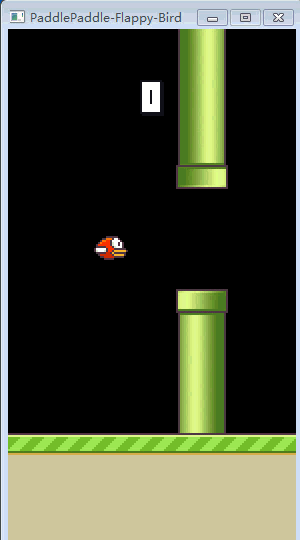Auto ByteScience AI### 模拟环境未经训练的小笨鸟

BirdEnv.py 继承自 gym.Env，实现了 init、reset、reward、render 等标准接口。init 函数，用于加载图片、声音等外部文件，并初始化得分、小鸟位置、上下边缘、水管位置等环境信息：

``````def __init__(self):
if not hasattr(self,'IMAGES'):
print('InitGame!')
self.beforeInit()
self.score = self.playerIndex = self.loopIter = 0
self.playerx = int(SCREENWIDTH * 0.3)
self.playery = int((SCREENHEIGHT - self.PLAYER_HEIGHT) / 2.25)
self.baseShift = self.IMAGES['base'].get_width() - self.BACKGROUND_WIDTH
newPipe1 = getRandomPipe(self.PIPE_HEIGHT)
newPipe2 = getRandomPipe(self.PIPE_HEIGHT)
#...other code``````

step 函数，执行两个动作，0 表示不采取行动（小鸟会自动下落），1 表示飞翔；step 函数有四个返回值，image_data 表示当前状态，也就是游戏画面，reward 表示本次 step 的即时奖励，terminal 表示是否是吸收状态，{} 表示其他信息：

``````def step(self, input_action=0):
pygame.event.pump()
reward = 0.1
terminal = False
if input_action == 1:
if self.playery > -2 * self.PLAYER_HEIGHT:
self.playerVelY = self.playerFlapAcc
self.playerFlapped = True
#...other code

image_data=self.render()
return image_data, reward, terminal,{}``````

``````#飞行一段距离,奖励+0.1
reward = 0.1
#...other code

playerMidPos = self.playerx + self.PLAYER_WIDTH / 2
for pipe in self.upperPipes:
pipeMidPos = pipe['x'] + self.PIPE_WIDTH / 2
#穿过一个柱子奖励加1
if pipeMidPos <= playerMidPos < pipeMidPos + 4:
self.score += 1
reward = self.reward(1)
#...other code

if isCrash:
#撞到边缘或者撞到柱子,结束,并且奖励为-1
terminal = True
reward = self.reward(-1)``````

reward 函数，返回即时奖励 r：

``````def reward(self,r):
return r``````

reset 函数，调用 init，并执行一次飞翔操作，返回 observation,reward,isOver：

``````def reset(self,mode='train'):
self.__init__()
self.mode=mode
action0 = 1
observation, reward, isOver,_ = self.step(action0)
return observation,reward,isOver
``````

render 函数，渲染游戏界面，并返回当前画面：

``````def render(self):
image_data = pygame.surfarray.array3d(pygame.display.get_surface())
pygame.display.update()
self.FPSCLOCK.tick(FPS)
return image_data``````

### DQN的发展过程

DQN 的进化历史可谓源远流长，从最开始 Bellman 在 1956 年提出的动态规划，到后来 Watkins 在 1989 年提出的的 Q-learning，再到 DeepMind 的 Nature-2015 稳定版，最后到 Dueling DQN、Priority Replay Memory、Parameter Noise 等优化算法，横跨整整一个甲子，凝聚了无数专家、教授们的心血。如今的我们站在先贤们的肩膀上，从以下角度逐步分析：

• 贝尔曼（最优）方程与 VQ 树

• Q-learning

• 参数化逼近

• DQN 算法框架

### 贝尔曼 (最优) 方程与VQ树

• S 状态集合，维度为 1×|S|

• A 动作集合，维度为 1×|A|

• P 状态转移概率矩阵，经常写成，其维度为 |S|×|A|×|S|

• R 回报函数，如果依赖于状态值函数 V，维度为 1×|S|，如果依赖于状态-动作值函数 Q，则维度为 |S|×|A|

• γ 折扣因子，用来计算带折扣的累计回报 G(t)，维度为 1

S、A、R、γ 均不难理解，可能部分同学对有疑问——既然 S 和 A 确定了，下一个状态 S' 不是也确定了吗？为什么会有概率转移矩阵呢？

1.恒等于 1.0 的情况。如图 1 所示，也就是上一次我们在策略梯度算法中所使用的迷宫，假设机器人处于左上角，这时候你命令机器人向右走，那么他转移到红框所示位置的概率就是 1.0，不会有任何异议：图1. 迷宫寻宝2.不等于 1.0 的情况。假设现在我们下一个飞行棋，如图 2 所示。有两种骰子，第一种是普通的正方体骰子，可以投出 1~6，第二种是正四面体的骰子，可以投出 1~4。现在飞机处于红框所示的位置，现在我们选择投掷第二种骰子这个动作，由于骰子本身具有均匀随机性，所以飞机转移到终点的概率仅仅是 0.25。这就说明，在某些环境中，给定 S、A 的情况下，转移到具体哪一个 S' 其实是不确定的：图2. 飞行棋

• 策略 π(a∣s)，维度为 |S|×|A|

• 状态值函数，维度为 1×|S|，表示当智能体采用策略 π 时，累积回报在状态 s 处的期望值：图3 状态值函数• 状态-行为值函数，也叫状态-动作值函数，维度为 |S|×|A|，表示当智能体采取策略 π 时，累计回报在状态 s 处并执行动作 a 时的期望值：• 将总问题划分为 k 个子问题，递归求解这些子问题，然后将子问题进行合并，得到总问题的最优解；对于重复的子问题，我们可以将他们进行缓存（记忆搜索 MemorySearch，请回忆 f(n)=f(n-1)+f(n-2) 这个递归程序）；

• 计算最小的子问题，合并这些子问题产生一个更大的子问题，不断的自底向上计算，随着子问题的规模越来越大，我们会得到最终的总问题的最优解（打表 DP，请回忆杨辉三角中的 dp[i-1,j-1]+dp[i-1,j]=dp[i,j]）。

• 处在状态 s 时，由于有策略 π 的存在，故可以把状态值函数 v 展开成以下形式：图5. v展开成q

• 处在状态 s、并执行动作 a，可以把状态-行为值函数 q 展开成以下形式：图6. q展开成v图7. V-Q树图8. 状态值函数v自身的递归展开式图9. 状态-行为值函数q自身的递归展开式

• 最优状态值函数，表示在所有策略中最大的值函数，即：图10. 最优状态值函数

• 最优状态-行为值函数，表示在所有策略中最大的状态-行为值函数：图11. 最优状态-行为值函数图12. v的贝尔曼最优方程图13. q的贝尔曼最优方程图14. 算法收敛时候的策略π图15. 动态规划方法的解空间搜索过程

### Q-learning图16. MC系列方法的解空间搜索过程

1. 使用作为训练标签，其本身就是值函数准确的无偏估计。但是，这也正是它的缺点，因为 MC 方法会经历很多随机的状态和动作，使得每次得到的 G(t) 随机性很大，具有很高的方差。

2. 由于采用的是一条道走到黑的方式从根节点遍历到叶子节点，所以必须要等到 episode 结束才能进行训练，而且每轮 episode 产生的数据只训练一次，每轮 episode 产生数据的 batch-size 还不一定相同，所以在训练过程中，MC 方法的 loss 函数（或者 TD-Error）的波动幅度较大，而数据利用效率不高。

1. TD(0) 算法，只向后估计一个 step。其值函数更新公式为：图17. TD(0)算法的更新公式

2. Sarsa(λ) 算法，向后估计 n 步，n 为有限值，还有一个衰减因子 λ。其值函数的更新公式为：图18. Sarsa(λ)算法的更新公式图19.的计算方法图20. TD方法的解空间搜索过程图21. Q-learning算法的学习公式

``````#define maxEpisode=65535 //定义最大迭代轮数
#define maxStep=1024 //定义每一轮最多走多少步
initialize Q_table[|S|,|A|] //初始化Q矩阵
for i in range(0,maxEpisode):
s=env.reset()  //初始化状态s
for j in range(0,maxStep):
//用ε-greedy策略在s行选一个动作a
choose action a using ε-greedy from Q_table[s]
s',R,terminal,_=env.step(a) //执行动作a,得到下一个状态s',奖励R，是否结束terminal
max_s_prime_action=np.max(Q_table[s',:]) //选s'对应的最大行为值函数
td=R+γ*max_s_prime_action //计算TD目标
Q_table[s,a]= Q_table[s,a]+α*(td-Q_table[s,a]) //学习Q(s,a)的值
s=s' //更新s，注意，和sarsa算法不同，这里的a不用更新
if terminal:
break
``````

Q-learning 是一种优秀的算法，不仅简单直观，而且平均速度比 MC 快。在 DRL 未出现之前，它在强化学习中的地位，差不多可以媲美 SVM 在机器学习中的地位。

### 参数化逼近图22. Q-Learning的优化目标图23. 带参数的优化目标图24. loss函数的梯度下降公式图25. 含有目标网络参数θ'的梯度下降公式 至此，DQN 的 Loss 函数、梯度下降公式推导完毕。

### DQN算法框架

DQN 的基本网络结构如下：图26. DQN的基本网络结构

1. 与参数 θ 做线性运算 (wx+b) 的仅仅是输入状态 s，这一步没有动作 a 的参与；

2. output_1 的维度为 |A|，表示神经网络 Q(s,θ) 的输出；

3. 输入动作 a 是 one-hot，与 output_1 作哈达马积后产生的 output_2 是一个数字，作为损失函数中的 Q(s,a|θ)，也就是 y。

``````#Deep-Q-Network,Nature 2015 version

#定义为一个双端队列D，作为经验回放区域，最大长度为max_size
Initialize replay_memory D as a deque,mas_size=50000

#初始化状态-行为值函数Q的神经网络，权值随机
Initialize action-value function Q(s,a|θ) as Neural Network with random-weights-initializer

#初始化TD目标网络，初始权值和θ相等
Initialize target action-value function Q(s,a|θ) with weights θ'=θ

#迭代max_episode个轮次
for episode in range(0,max_episode=65535):

#重置环境env，得到初始状态s
s=env.reset()

#循环事件的每一步，最多迭代max_step_limit个step
for step in range(0,max_step_limit=1024):

#通过ε-greedy的方式选出一个动作action
With probability ε select a random action a or select a=argmax(Q(s,θ))

#在env中执行动作a，得到下一个状态s'，奖励R,是否终止terminal
s',R,terminal,_=env.step(a)

#将五元组(s,a,s',R,terminal)压进队尾

#如果队列满，弹出队头元素
if D.isFull():
D.removeFirst()

#更新状态s
s=s'

#从队列中进行随机采样
batch_experience[s,a,s',R,terminal]=random_select(D,batch_size=32)

#计算TD目标
target = R + γ*(1- terminal) * np.max(Q(s',θ'))

θ=θ+α*(target-Q(s,a|θ))▽Q(s,a|θ)

#每隔C步，同步θ与θ'的权值
Every C steps set θ'=θ

#是否结束
if terminal：
break ``````图27. Atari游戏的CNN网络结构图28. 神经网络的具体参数

### 代码实现

1. BirdEnv.py，环境；上文已经分析过了。

2. BirdModel.py，神经网络模型；使用三层 CNN+两层 FC，CNN 的 padding 方式都是 valid，最后输出状态-行为值函数 Q，维度为 |A|。注意输入图片归一化，并按照官方模板填入代码：

``````class BirdModel(Model):
def __init__(self, act_dim):
self.act_dim = act_dim
p_valid=0
self.conv1 = layers.conv2d(
self.conv2 = layers.conv2d(
self.conv3 = layers.conv2d(
self.fc0=layers.fc(size=512)
self.fc1 = layers.fc(size=act_dim)

def value(self, obs):
#输入归一化
obs = obs / 255.0
out = self.conv1(obs)
out = self.conv2(out)
out = self.conv3(out)
out = layers.flatten(out, axis=1)
out = self.fc0(out)
out = self.fc1(out)
return out``````

3. dqn.py，算法层；官方仓库已经提供好了，我们无需自己再写，直接复用算法库（parl.algorithms）里边的 DQN 算法即可。

define_learn 函数，用于神经网络的学习。接收 [状态 obs, 动作 action, 即时奖励 reward, 下一个状态 next_obs, 是否终止 terminal] 这样一个五元组，代码实现如下：

``````#根据obs以及参数θ计算状态-行为值函数pred_value，对应伪代码中的Q(s,θ)
pred_value = self.model.value(obs)

#根据next_obs以及参数θ'计算目标网络的状态-行为值函数next_pred_value，对应伪代码中的Q(s',θ')
next_pred_value = self.target_model.value(next_obs)

best_v = layers.reduce_max(next_pred_value, dim=1)

#计算TD目标
target = reward + (1.0 - layers.cast(terminal, dtype='float32')) * self.gamma * best_v

#输入的动作action与pred_value作哈达玛积，选出要评估的状态-行为值函数pred_action_value，对应伪代码中的 Q(s,a|θ)
action_onehot = layers.one_hot(action, self.action_dim)
action_onehot = layers.cast(action_onehot, dtype='float32')
pred_action_value = layers.reduce_sum(layers.elementwise_mul(action_onehot, pred_value), dim=1)

#mse以及梯度下降，对应伪代码中的θ=θ+α*(target-Q(s,a|θ))▽Q(s,a|θ)
cost = layers.square_error_cost(pred_action_value, target)
cost = layers.reduce_mean(cost)
optimizer.minimize(cost)``````

sync_target 函数用于同步网络参数

``````def sync_target(self, gpu_id):
""" sync parameters of self.target_model with self.model
"""
self.model.sync_params_to(self.target_model, gpu_id=gpu_id)``````

4. BirdAgent.py，智能体。其中，build_program 函数封装了 algorithm 中的 define_predict 和 define_learn，sample 函数以 ε-greedy 策略选择动作，predict 函数以 100% 贪心的策略选择 argmax 动作，learn 函数接收五元组 (obs, act, reward, next_obs, terminal) 完成学习功能，这些函数和 Policy-Gradient 的写法类似。

``````#保存模型
def save_params(self, learnDir,predictDir):
fluid.io.save_params(
executor=self.fluid_executor,
dirname=learnDir,
main_program=self.learn_programs)
fluid.io.save_params(
executor=self.fluid_executor,
dirname=predictDir,
main_program=self.predict_programs)

#加载模型
executor=self.fluid_executor,
dirname=learnDir,
main_program=self.learn_programs)
executor=self.fluid_executor,
dirname=predictDir,
main_program=self.predict_programs) ``````

``````#每训练多少步更新target网络,超参数可调
self.update_target_steps = 5000

#初始探索概率ε,超参数可微调
self.exploration = 0.8

#每步探索的衰减程度,超参数可微调
self.exploration_dacay=1e-6

#最小探索概率,超参数可微调
self.min_exploration=0.05
``````

5. replay_memory.py，经验回放单元。双端队列 _context 是一个滑动窗口，用来记录最近 3 帧（再加上新产生的 1 帧就是 4 帧）；state、action、reward 等用 numpy 数组存储，因为 numpy 的功能比双端队列更丰富，max_size 表示 replay_memory 的最大容量：

``````self.state = np.zeros((self.max_size, ) + state_shape, dtype='int32')
self.action = np.zeros((self.max_size, ), dtype='int32')
self.reward = np.zeros((self.max_size, ), dtype='float32')
self.isOver = np.zeros((self.max_size, ), dtype='bool')
#_context是一个滑动窗口,长度永远保持3
self._context = deque(maxlen=context_len - 1)
``````

6. Train_Test_Working_Flow.py，训练与测试，让环境 evn 和智能体 agent 进行交互。最重要的就是 run_train_episode 函数，体现了 DQN 的主要逻辑，重点分析注释部分与 DQN 伪代码的对应关系，其他都是编程细节：

`````` #训练一个episode
def run_train_episode(env, agent, rpm):
global trainEpisode
global meanReward
total_reward = 0
all_cost = []
#重置环境
state,_, __ = env.reset()
step = 0
#循环每一步
while True:
context = rpm.recent_state()
context.append(resizeBirdrToAtari(state))
context = np.stack(context, axis=0)
#用ε-greedy的方式选一个动作
action = agent.sample(context)
#执行动作
next_state, reward, isOver,_ = env.step(action)
step += 1
#存入replay_buffer
rpm.append(Experience(resizeBirdrToAtari(state), action, reward, isOver))
if rpm.size() > MEMORY_WARMUP_SIZE:
if step % UPDATE_FREQ == 0:
#从replay_buffer中随机采样
batch_all_state, batch_action, batch_reward, batch_isOver = rpm.sample_batch(batchSize)
batch_state = batch_all_state[:, :CONTEXT_LEN, :, :]
batch_next_state = batch_all_state[:, 1:, :, :]
#执行SGD,训练参数θ
cost=agent.learn(batch_state,batch_action, batch_reward,batch_next_state, batch_isOver)
all_cost.append(float(cost))
total_reward += reward
state = next_state
if isOver or step>=MAX_Step_Limit:
break
if all_cost:
trainEpisode+=1
#以滑动平均的方式打印平均奖励
meanReward=meanReward+(total_reward-meanReward)/trainEpisode
print('\n trainEpisode:{},total_reward:{:.2f}, meanReward:{:.2f} mean_cost:{:.3f}'\
.format(trainEpisode,total_reward, meanReward,np.mean(all_cost)))
return total_reward, step
``````

``````#充满replay-memory,使其达到warm-up-size才开始训练
MEMORY_WARMUP_SIZE = MEMORY_SIZE//20

##一轮episode最多执行多少次step，不然小鸟会无限制的飞下去,相当于gym.env中的_max_episode_steps属性
MAX_Step_Limit=int(1<<12)

#用一个双端队列记录最近16次episode的平均奖励
avgQueue=deque(maxlen=16)
``````

``````#衰减因子
GAMMA = 0.99

#学习率
LEARNING_RATE = 1e-3 * 0.5

#记录日志的频率
log_freq=10``````

main 函数在这里，输入 train 训练网络，输入 test 进行测试：

``````if __name__ == '__main__':
print("train or test ?")
mode=input()
print(mode)
if mode=='train':
train()
elif mode=='test':
test()
else:
print('Invalid input!')
``````图29. 模型训练的输出日志图30. 最近16次平均奖励变化曲线训练完的FlappyBird

#### 参考文献

 Bellman, R.E. & Dreyfus, S.E. (1962). Applied dynamic programming. RAND Corporation.

 Sutton, R.S. (1988). Learning to predict by the methods of temporal difference.Machine Learning, 3, pp. 9–44.

 V. Mnih, K. Kavukcuoglu, D. Silver, A. A. Rusu, et al., "Human-level control through deep reinforcement learning," Nature, vol. 518(7540), pp. 529-533, 2015.

 https://leetcode.com/problems/climbing-stairs/

 https://leetcode.com/problems/pascals-triangle-ii/

 https://github.com/yenchenlin/DeepLearningFlappyBird

 https://github.com/MorvanZhou/Reinforcement-learning-with-tensorflowPaperWeekly

DeepMind机构

https://deepmind.com/

（人工）神经网络是一种起源于 20 世纪 50 年代的监督式机器学习模型，那时候研究者构想了「感知器（perceptron）」的想法。这一领域的研究者通常被称为「联结主义者（Connectionist）」，因为这种模型模拟了人脑的功能。神经网络模型通常是通过反向传播算法应用梯度下降训练的。目前神经网络有两大主要类型，它们都是前馈神经网络：卷积神经网络（CNN）和循环神经网络（RNN），其中 RNN 又包含长短期记忆（LSTM）、门控循环单元（GRU）等等。深度学习是一种主要应用于神经网络帮助其取得更好结果的技术。尽管神经网络主要用于监督学习，但也有一些为无监督学习设计的变体，比如自动编码器和生成对抗网络（GAN）。

Q学习技术

Q学习是一种用于机器学习的强化学习技术。 Q-Learning的目标是学习一种策略，告诉智能体在什么情况下要采取什么行动。 它不需要对环境建模，可以处理随机转换和奖励的问题，而无需进行调整。